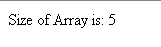Tutorials

# JSP Array Length

JSP Array Length is performed when you want to compute the length of array in JSP.

JSP Array Length is performed when you want to compute the length of array in JSP.

# JSP Array Length

JSP Array Length is performed when you want to compute the length of array in JSP.

Understand with Example

The Tutorial illustrate an example from 'JSP Array Length'. To understand and grasp this example we create a page directive that include the language type is Java. You can see in the example that we create a Scriptlets that include an array of integer. To print the size of array we  use out.print in JSP.

array.length( )  : This method is used to display the length of array.

Here is the code of jspArrayLength.jsp

 <%@ page language="java"%> <% int array[]={2,4,6,8,10}; out.print("Size of Array is: "+array.length); %>

Output will be displayed as: